Select Page

# A New Constant

### Built into the Alef-BetIn our article The Creator’s Smoking Gun we showed that while the Hebrew Alef-bet is assigned its values based on the exponential scale 1.313x, the 22 Names of the Alef-bet are repeatedly and fractally based on the Phi (φ) proportion. It began with (2 x 1313) or the 2626, the Even portion of the total 4248-value of the 22 Names, that is in the ratio of 2626/4248 = .618173 = 1/Phi (φ) and continued with 1622 being approximately 1618 or Phi (φ), and the ratio of the Even to Odd Names or 2626/1622 being equal to 1.618 or Phi (φ) again.

There were a dozen other connections in this 11 Odd and 11 Even Names split down the middle that align with the perfect Golden Mean proportions. Other examples are the ratio of the total 22 Names to the 11 Odd Names or 4248/1622 = 2.618 or Phi (φ)2 and the ratio of the difference between the 2 sets of Odd and Even Names to the whole, or 4248/1004 = 4.2311, approximately Phi (φ)3.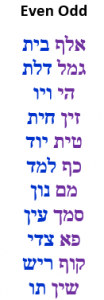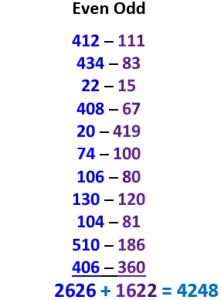Those 22 Names each have an initial and these 22 initials make up the 22 letters of the Alef-Bet. As we saw, those 22 initials split into the same Odd and Even sets and also gave astonishing results, such as the 11 Odd letters total of 625 being exactly equal to the square root of all the words, letters, and verses in the Torah: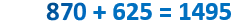Those 22 Names also have backs to their Names and these 22 sets of backs are likewise divisible into the same 11 Odd and 11 Even sets.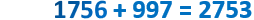The ratios of the Odd initials (fronts) and backs sets to the whole 11 Odd Names (625/997, 997/1622) is also very close to the Phi (φ), while the ratios of the Even initials (fronts) and backs sets to the whole 11 Even Names (870/2626, 1756/2626) is in the kabbalistic (.333, .666., .999) proportion, or as Tesla called it, the secret (369) key to the universe.

Even the sum of the total Torah occurrences of those 22 letters when split into the same 11 Odd and 11 Even sets are split very nearly into the Phi (φ) proportion, off by only .008.

### It Starts with the Singularity of 42

Nonetheless, these are not the only secret patterns and proportions hidden in this simplest of splits of the Alef-Bet, the interface language with the numeric language of the Cosmos. The ratio of all 22 sets of backs to the 22 Names is 2753/4248 = .6480 and 6480 is not only the square root of 42 or 6.480, but like Phi (φ) is it a proportion deeply ingrained in the fabric of the Torah and the Cosmos.

Plato valued it so much that he assigned the 6480o of the 5th Platonic Solid, the 12-pentagonal sided dodecahedron, to the aether that pervades the cosmos on all levels.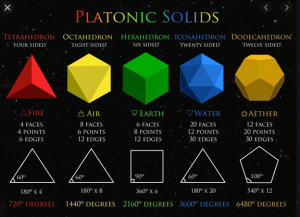In terms of the Torah, the respective quantitative totals (390,625 and 401,273 or 58 and 248,000/φ) of the Torah’s 3 and 5 main elements both align directly with the same 6480 or √42.  All that is needed is to apply the simple number theory application of the product of the digits in the equation divided by the sum of the digits.

To begin with, the sum of the digits in (401273 + 390625) is 42, and the full P/S gematria cipher of 390,625 is 1620/25 = 64.8, which references √42.

Then the P/S of (390,625 and 401,273) together is 272160/42 = 6480, or exactly 100 times the result of previous equation.  This resultant 6480 is also the 80th Pronic Number or 80 x 81, as in the (80,00025) words in the Torah.

What makes this alignment more astounding is that the cube root of the product of the digits in (390,625 and 401,273), or 272160, is 64.80

Then applying the cipher again to this result, product of the digits in the resultant value 272160 is 168 or (4 x 42). Thus we get 168/6 or (4 x 42)/(4 + 2) = 18, like the sum of the digits in 272160.

The difference between the two sets of Torah elements is (401273390625) = 223 , which is 10,648 and (648 x 10) = 6480 again. That difference represents the rows and columns in the Torah and those 10648 rows and columns plus the 183 or 5832 verses equal (223 + 183) = 16480, or (104 + 6480).

It always come down to 42, like the 42 rows per column.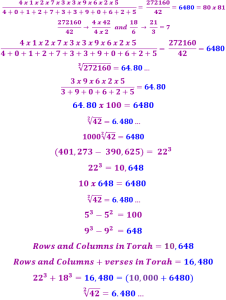### The Great Precession

As for the structure of the Cosmos, the great precession of the Earth is a circle of 25,920 years. When we divide that into the 12 constellations or 12 lunar months in the Cosmic Wheel, we get an arc of 2160 years each, exactly one lunar diameter. Those 25,920 years are metaphysically equivalent to the 25,920 parts of a minute in 1 day (18 parts x 60 minutes x 24 hours). One (1) part of a minute equals 3.333 seconds.

So, if 25,920 years equals 1 days’ worth of parts of a minute, then each of those years is metaphysically equivalent to 1 part of a minute, or 3.333 seconds per year of the precession.

This is also equivalent to (2160 x 3.333…) = 7200.0 seconds per 1/12th or lunar period, aligning the Moon whose diameter is (2160/3 = 720 miles) with the 72 Triplets (3-letters sequences) concealed with the Torah. This is also analogous to the 72 square roots of those 72 Triplets that equal precisely 720.0.

On the other hand, (2160/3.333…) = 648.06480, which aligns the Moon and constellation passage with the square root of 42 and thus with the 14 Triplets of the 42-Letter Name that are also concealed within the Torah.  The Moon would appear to act as a balance between the 72 Triplets or Names and the 42-Letter Name. The ratio of the 18 parts of a minute to the second (3.333) and its inverse (1/3) align with the Moon (2160 miles) to form the balancing point between the 72 Triplets (Names) and the 42-Letter Name.

Of course, the Earth and the Sun play a part as well. The Earth’s precession is based on the tilt of its axis of (90o23.4o) = 66.6o. The diameter of the Sun divided by the 25,920-year precession is 864,000/25,920 = 33.333, matching the Sun’s Mass/Earth’s Mass ratio (333,000), which itself is 5 x 66,600 mph, the Earth’s Orbital speed around the Sun, or alternatively the Sun’s apparent orbit around the Earth.  The precession is a circumference, the Sun measurement is a diameter, and the orbital speed is traced along another circumference.

Moreover, the Sun’s diameter is to the Great Precession as the part of a minute is to the second.  Distance and Time aligned.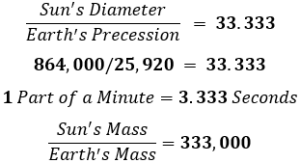The inverse of all these equations is equivalent to exactly 3 x 10n, in other words the Earth to the Sun and the Precession to the Sun and the second to the part of a minute. The structure of our solar system, which let us face it, is our entire universe can not be any simpler than that.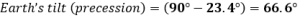The Sun’s diameter of 864,000 miles divided by Pi (π) equals 275019.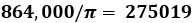The 25,920 years in the circle of the Great Precession divided by Pi (π) equals 3 x 2750.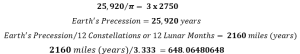Conversely, the Moon’s diameter is 1/3rd of 6480.  Again, simplicity.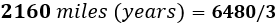## The Singularity of 42 and the Endless.

The Singularity of 42 is a deeper connection to the essence of the Cosmos than a Name of the Creator. It is a concept of the endless and it goes beyond any of the other Names and even beyond the various Names of 42 themselves. It is One with the Source and thus a Singularity like no other.

Spherical Time happens as a consequence of the interaction of the fields of Phi (φ) with Pi (π) and the vortex generated through them by the Source of 42. As we will see shortly the number 9 or 32 plays a significant role in the generation of the Primal Frequency 27.5. It is so important that the value 9 is found 26 times in the Torah like the value of the YHVH (יהוה), while the Hebrew word (תשע) for 9 is found 9 times in the Torah, and while there are 9 spaces that separate the paragraphs in the Torah, there are 9 sets of chronicles in the Torah.

The value 9 most notably interacts with the 42-Letter Name to produce the field of Pi (π).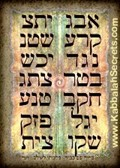When we divide the number 9 by the first level of the 42-Letter Name Matrix we get 9/.123049 = 73.141593 or 70 + Pi (3.1415926…). The fuller equation using the full 42 Letters is 9/.1230490273… = 73.14157771495…, which in addition to 70 and Pi (π), and the 9th sefira of Chochma (73) that defines the Torah’s first verse through the value of the 73rd Triangular Number, we have the allusion to the Cosmic Harmonic 273 built into the 42-Letter Name. There is also the year 5777 HC, and the sum of the 22 Letters of the Alef-bet, 1495. Then there is 73.14157771495…/2 which equals 36.5708 or the 365 Solar Days and the prophesied 70 years earlier than 5777/8, with the year 5708 HC, when Israel became a nation. Alternatively, the result displays the 365 Solar Days and the 708 value of the Upper 42-Letters of the Name YHVH (יהוה) that are so integral to the Cosmic Wheel. It is also the 708-value of “The Garden (הגן)” where we find the Tree-of-life.

It is off from precise Pi (π) by a minuscule 0.000475511534410972774603022149320519739105422392% and its inverse is 2,102.99840831142680368824376485618638050000784757688055 or simply 2103, as in the 210 years of exile and the 210-cubit height of the Tower of Truth. That degree of precision is more than NASA supposedly uses and enough to accurately traverse the Solar System.

Yet, before we see all that in 73.14157771495 we find the value 1577, which is the sofit value of Ein Sof (אין־סוף), the metaphysical Endless, the Infinite, G-d transcendent, the Pure Essence of G-d, the state of the Creator before all Creation. It is the state of existence before existence, the ultimate Source.

The concept of 42 truly does hold all the secrets of the universe.

### Spherical Time and the Great Precession

There are 3 similar numbers given for the Earth’s precession on its axis, the precession of the equinoxes. The first is 26,000 years. This cosmic declaration of the YHVH (יהוה) is obvious through the value 26 x 1000 (Binah). This 26,000-year value, though, may be more symbolic than actual, though the equation regarding the cycle of 54 portions in 5778 years or (5778 x 54)/12 = 26,001 portions, certainly is not.  We can ask why 26,001 and not the seemingly perfect 26,000 that man would have come up with? Like the Torah’s first verse at 2701, and the 42-Letter Name at 3701, the addition One (1) maybe the Creator’s calling card and an easy hint that Oneness and Love are all-important to releasing the power of the 54 portions and to conquering Spherical Time.

The second value given for this precession or great cycle in relation to the stars/constellations is 25,920 years. It is 80 years less than 26,000 as in the letter Pe (פ), and 81 years less than 26,001, as in the 81,000 words and word values. Together, they represent the 80th Pronic Number, 6480, the square root of 42. This has been the more precise scientific measure universally recognized until most recently. It has also been the Yuga cycle calculated in ancient India. It is also the measure of our clock. You see, 25,920 = 24 hours x 60 minutes x 18 parts of a minute, which is how we count time in Hebrew. The great cycle is thus equal to the parts of a minute in a single day.  One year of rotation for each part of a minute in a single day.  Time is elastic in the hyper-dimensions and our limited definition of a day is not the same as the Creator. A day, like a day of Creation can be 25,920 years long. And every fraction of a minute, every 3.333 seconds, is connected to an entire year within the precession or great cycle.As we have explained in previous articles and will do so in depth again in an upcoming one, the generation of Spherical Time comes from the spinning of a central Phi (φ) vortex upon a naturally drawn or virtual circle. It is the property of Phi (φ) that no two spiraled lines can ever cross that circle at the same point that make each time-path unique, and that allow for infinite generation. Each new time path is etched with every 137.5o turn of the Phi (φ) vortex upon the circle. Thus, in a single day of our time 25,920 years x 365.256 days x 24 hours x 60 minutes x 18 parts of a minute, 10,224,830,362 different time paths are generated.  That is with the Spherical Time clock turning once every 3.333 cosmic seconds, or 495o in 10 cosmic seconds. That 495o is equivalent to the sum of the values in the natural split of the first 18 letters of the Alef-bet.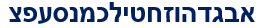The cosmos count time in the hierarchy of 12’s the same way we count in feet of 12 inches. The value 25,920 is equal to 12 x 2160, the diameter of the Moon, which is thus equivalent to 120 x 216, the age of Man times the sefira of Gevurah (216), Judgement. There are also 216 letters in the 72 Triplets. Indeed, the Great Precession advances 1o every 72 years.

Those 72 years can be thought of as a radian in the Great Cycle and if the Phi (φ)-clock turned its 495o or 1.375% in those 72 years. It would turn 495 rotations over the 25,920-year cycle.  Each of those 495o cycles equals 8.64 or 2.75π radians, and those 8.64 radians align with the 864,000 mi diameter of the Sun, and they both are thus aligned with the mysterious constant 27.5.

Over that 72-year span or 1o of the circle of the Great Precession, we can divide 72/2.75 = 26.1818 to get approximately 10 Phi (φ)2, and then dividing that result, 26.1818/π, gives us 8.333… or approximately 1/12, representing the universal constant of time (12).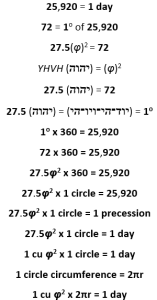What this means is that the 25,920-year Great Cycle is the measure of 1 unit of the constant 27.5 times Phi (φ)2 for each degree of the circle (cycle), with Phi (φ)2 as 2.61818, representing the relationship of 72/2.75:

### 27.5φ2 x 360 = 25,920

This mysterious constant (27.5) is space. The cycle is time.  Time is not a dimension. It is an interaction. Space and Time interact.  The circle is guided by the Pi (π) field. It is the interaction between the Phi (φ) field and the Pi (π) field that creates the infinite pathways of Spherical Time.

The Name YHVH (יהוה) has the numerical value 26 and when spelled-out in its highest form it becomes the more powerful Name YHVH (יוד־הי־ויו־הי) of numerical value 72, so based on what we have just learned the factor of expansion within that Holy Name is the constant 27.5. Like the individual letters, the Names of the letters or in this case the Name of Name is on a higher dimensional level than the Name or individual letters themselves. Thus, this expansion based on the constant 27.5 is a dimensional expansion. Alternatively, the Name YHVH (יהוה) is a dimensional contraction from the Name YHVH (יוד־הי־ויו־הי), as what occurs from going from the dimension of Binah to Zeir Anpin.

Binah to Zeir Anpin.

### The 22 Letters and Phi (φ)

Like the balancing act of the Moon, the Odd/Even split of the letters in the Alef-bet are split in proportions that align themselves with the 42-Letter Name and the 72 Names.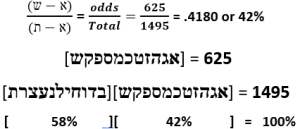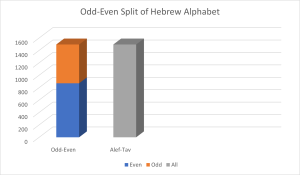This is the proportion of the smaller segment (625) in terms of numerical value to the total value of the Alef-bet (1495), in other words, the ratio of the odd ordinal letters to the total letters. Therefore, the 11 letter Alef-Shin (ש – א) segment of the Alef-bet has a total value equal to 42% of the whole 22 letter Alef – Tav (ת – א), and the Alef-Shin (ש – א) Odd side of the alphabet has a total value equal to 72% of the Even Bet – Tav (ת – ב) side of the split.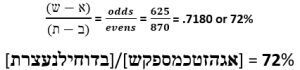The Odd/Even split of the letters in the Alef-bet are off by 54 from the exact Phi (φ) proportion split. When the values are shifted by 54 units the .4180 becomes .6180 and the .7180 also becomes .6180 or 1/ Phi (φ).

If we were to apply the Phi (φ) proportion to the 1495 total value of the alef-bet, the subordinate values would be split into 924 + 571 = 1495.

1495 x .6180 = 924

924  x  .6180 = 571

1495

Comparing the Phi (φ) proportioned Alef-bet to the Odd-Even split we can see that it is an exact shift of 54 units from Phi (φ) to the Odd/Even split.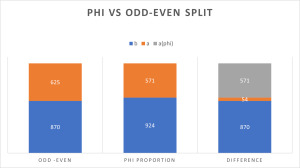625 – 54 = 571

870 + 54 = 924

When the Odd/Even split in the Alef-bet is arranged in a 360o circle, that shift of 54 units becomes a shift of exactly 13o and because circles are actually wave functions.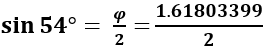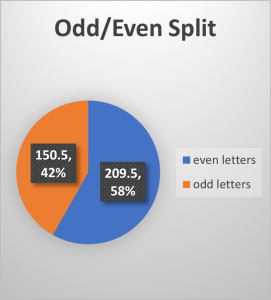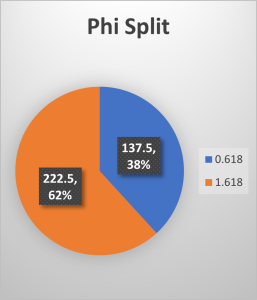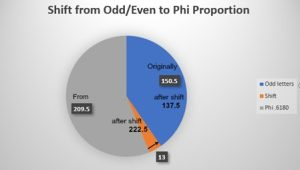When we multiply love and Oneness (13) by the proportional time value (54), we get (13 x 54) = 702, the numerical value of Shabbat (שבת), the 13th even Pronic number and 26th overall one. It is our connection to Binah, a period of time and space beyond the limits of our Spherical Time bubble and beyond the 6 dimensions of Zeir Anpin that create physicality.

When we add 702 to 5778 as the Great Shabbat that is prophesied to follow the 5778 years of pure physicality, we get: (5778 + 702) = 6480, which also equals (7200720). Once again, the square root of 42 at the heart of the Torah and Spherical Time.

In terms of understanding this in the paradigm of the cosmic simulation, the prophesied 7th millennium of the Great Shabbat is the next level of Consciousness. It is the state of Moshiach Consciousness that comes to those that awakened and did their spiritual work before the great reset. It is another level of Understanding for our souls, another simulation in the mind of the Creator, but much more ethereal and less physical and devoid of evil and the evil inclination. The work there is spiritually more challenging because we do not have physical temptations to fight off, so it is more difficult to tell if we are on the right track. There, we must develop our other senses. This is why we start down here in Malchut, the physical simulation.

The next level of simulation is harder to awaken from because it is so wonderful and blissful why would we want to. It is like the town of Middleton in The Good Witch a veritable paradise where everyone thrives and grows spiritually, though not an ounce on their waistlines in spite of consuming copious amounts of tantalizing baked goods and delicious comfort food.  It is also where positive white magic, sage advice, and wondrous gifts are doled out lovingly and freely to nudge people along on their path. In other words, the opposite of our simulation.

And while the 7200 seconds in one Moon or 1/12th arc of the Precession is 120 minutes, the value 6480 = 120 x 54, which is the age of Man (120 years) times the annual cycle of the Torah, in other words 6480 Torah portions allotted per person.

So, which came first there being 54 portions in the Torah or 648.0/12 being equal to 54, the √42/12 = 54?

Those 7200 seconds in one Moon or 1/12th of the Precession divided by 6480 or the square root of 42 is 11.11, as in what is commonly called the Angelic Number. It is also the 671st minute of every 720 minutes in every half day or 12-hour period, like the value of the Name Adonai (אדני) when filled in (אלף־דלת־נון־יוד) and like the 671 million mph speed of light.

It is a time (11:11) and a number (11.11), like the Alef-bet, split 11 and 11. It is also One (1) and the balancing point between the 72 Names (Triplets) and the 42-Letter Name. It is a sign we are in alignment with the Cosmos and open to enlightenment. It is like Phi (ϕ), the cosmic balance that is equal to 1 + 1/(1 + 1…) and to  √(1 + √(1 + √(1 + √(1 +…).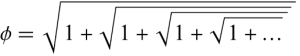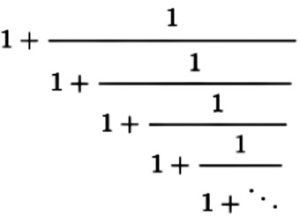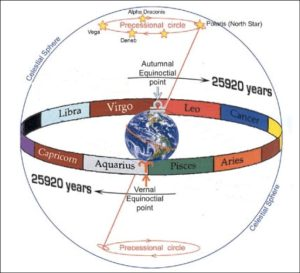This is the same Phi (φ) that we see expressed in the circle as the angle 137.5o, which is exactly half the 275.0 figure in the equations of the Sun’s diameter (864,000 mi) divided by Pi (π) = 275019, and in the 25,920 years of the circular Great precession divided by Pi (π) = 3 x 2750.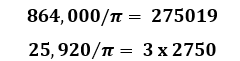This same figure of 2750 or 27.5 appears in conjunction with the square root of 42 as a ratio that gives us 4.24, Moshiach Ben David, the Cosmic Consciousness, as found in the Spherical Time circumference (36304.24470).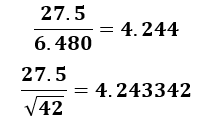This equation must cosmically and metaphysically and conceptually be very important.

It always comes down to 42. And like the 3342 in the result of the equation above, there are 42 letters in the 11 sefirot (dimensions) of the Tree-of-Life, whose total value is 3342, the same as 13 of the 14 Triplets in the 42-Letter Name that also equal (3701359) = 3342. Meanwhile, the first 42 letters of the Torah equal 3842, which is 500 more than 3342, as in the value of final Triplet (צית) in the 42-Letter Name. The first and last letters of those 42 letters of the 11 sefirot are Caf (כ) and Tav (ת), that according to Abraham Avinu, represent the Sun and the Moon with their combined numerical value of 420.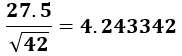This simple equation brings together 3 Primal components of Cosmic Consciousness. Moreover, the initials in those 11 sefirot equal 550 or (20 x 27.5).

This is all very odd because as we saw above the backs of the Names of the 22 Hebrew letters equal 2753, while the full Names of the Hebrew Letters equal 4248.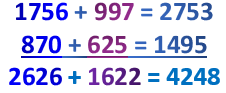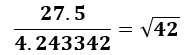By rearranging this core equation, we see that the backs of the Names of the 22 Hebrew letters divided by the full Names of the Hebrew Letters equal, in other words the ration of the Back of the 22 Names to the Names themselves is 2753/4248 = 10042.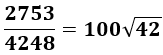If this key core arrangement between the geometry of the Cosmos, the structure of the Torah, and the interface language of the Alef-bet were not perplexing enough we see it again in the sum of the ordinal values of the 27 Hebrew letters, 378 divided by the Phi (φ) angle (137.5o) or 378/13.75 = 27.5. This is the same 378 as in the Magical Essential Cube of Creation of 42’s total value 378, which is also the same as “His Name is One.” This also works out to 27.52/2 = 378.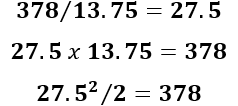Another odd alignment between the number 27.5, the Spherical Time equations, and the Alef-bet is found with the value 28, as in the 28 letters in the Torah’s first verse. We know the Alef-bet is based on the exponential scale 1.313x and when we raise 281.313 and divide by 27.5, we get 2.889 as in the midpoint in the Spherical Time radius, and the midpoint in King David’s 70-year life, 2889 HC.

And whichever way that these equations are cosmically connected, Phi (φ)/28 = .0577870, as the in the Spherical Time radius, 5778.There is a whole slew of extraordinary findings to be added using this simple formula involving the exponent 1.313 and the divisor 27.5, including one that involves the value 80, as in the 17th letter Pe (פ), which we know has a special function in the Torah due to its specific 4805 occurrences out of the 304805 total letters and due to their 6202 total value. Applying the same formula, we get 801.313 / 27.5 = 11.466, as in the exact square miles of the land of Israel today.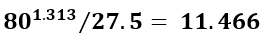The Name Israel (ישראל) is encoded in the Creation story of the Torah’s first chapter and is found there every 7th letter, while the Creator saw things as “good (טוב)” of numerical value 17, seven (7) times in that same chapter.  While 17 equals exactly 27.5/φ, and equals (27.5212/42), with the new formula we get 171.313 / 27.5 = 1.500, as in YH (יה) or 15 and as in the highest word value in the Torah, 1500, or 5 more than the 1495 value of the 22 letters.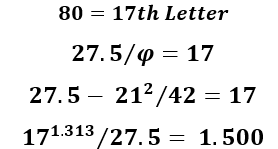We have already proven that the Alef-bet was meant to fit a curve based on 1.313 and that the variations from this curve follow a wave function.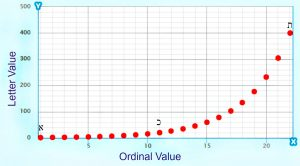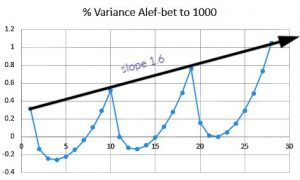Then when we add the absolute value for all 27 percentage variances, they equal 6.1823 or approximately 1/Phi (φ) or .618033….  As it turns out, the 21st letter, Shin (ש) that represents the 21 letters that total exactly 300,000 in the Torah, has a variance off its nominative value (300) of 4.48033… or precisely 1.70000… less than 1/Phi (φ), as in 17φ = 27.5. What is even more astonishing is that the letter Shin (ש) variance/10 plus 1/Phi (φ) or (.448033 + .618033) = 1.066066 and 66066 is the 363rd Triangular Number with 363 being the numerical value of H’Moshiach, as in the Spherical Time circumference (36304.24470).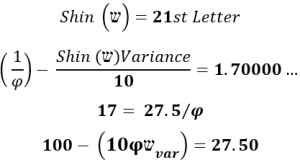I suppose it is fitting then that 21 x 1.313 = 27.573 and that the cumulative value of all 21 results of the curve function y = x1.313 through the letter Shin (ש) equals 1273.066066, as in the Cosmic Harmonic 1.273 and the 363rd Triangular Number again. Funny how the Cosmos worked 21 into the equation (27.5212/42) = 17 = 27.5/φ and into the split of the Torah letters.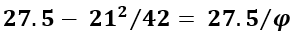One of the simplest connections, though, to the Alef-bet and the 22 Names is that the sum of the 22 integers plus 22 or (253 + 22) = 275.

### Another Way to Split the Alef-bet.

There is a more natural way, though, to split the Alef-bet into the 1/3 and 2/3 proportions that the Kabbalists use, including Rav Ashlag who as we explained earlier, used it to calculate the time of Moshiach as the period bounding 5778. If we separate the Alef-bet into the first 18 letters and the final 4 letters, in other words the single and double-digit letter values juxtaposed to the triple-digit letter values, we get a split of 495 and 1000.  This works out to .3311 and .6688, off from true .3333 and .6666 by a miniscule yet telling, .0022 and .0028 amounts, which is pretty much negligible for the most natural sequential split imaginable.  Oddly, when we take their ordinal values, the proportion is almost exactly reversed with the 18 letters equal to 171 out of a total 253, and the 4 last letters equal to 82. Yet what makes this truly interesting is that the complete value of those first 18 letters is (495 + 171) = 666.

One-third or .333 of the Alef-bet in terms of value equals exactly 666. And while 18 is .666 of the full 27 letters, it is not of this set of 22 in their standard form. That 18 = (6 + 6 + 6) adds to the mystique but does not answer how or why the Creator built this into the interface language of the Cosmos, the same language that is fully integrated into Phi (φ).

The 4 other letters that total 1000 have a complete value of (1000 + 82) and an average value of (1082/4) = 270.5, as in 27.5 and as in 4 more than the value of the Torah’s first verse, 2701.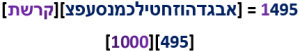Those first 18 letters match the 495o that are turned in the Spherical Time paradigm every 10 seconds.

We should note here that when we add 18 to 82 and conversely 4 to 171, we get 100 and 175 respectively, two key ages in the life of Abraham, as specifically delineated in the Torah. Abraham was 100 when Sarah gave birth to Isaac and he lived another 75 years to 175.

Also, even though we know Abraham was 70 during the Covenant of Halves in 2018 HC at the beginning of the 430-years of exile that ended in 2448 HC, the Torah uncharacteristically mentions he was 75 when he left his home in Charan, meaning his journey would last 100 years. Everything in the Torah is elucidated with multiple purposes in mind.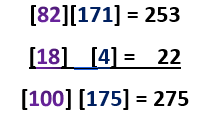Together the two splits in the alef-bet equal (100 + 175) = 275, which is independently derivative of the fact that there are 22 letters. The 22 letters plus the sum of their ordinal value, which is Σ (1 22) = 253, gives us (22 + 253) = 275. The proportion 100 is 36.36363% of 275, as in the numerical value of H’Moshiach (363), and 175 is 63.636363% of 275.

The Cosmic Consciousness was deftly woven into the design of the Alef-bet.

When we add the standard values to the above equation we get (100 + 1000) = 1100, which equals (40 x 27.5) and (175 + 495) = 670, as in the 670 paragraphs in the Torah and 10 times the value of Binah (67). Together, the 22 letters equate to (1100 + 670) = 1770 versus the standard value of 1775 for the 5 final letters, which are equated by the kabbalistic tradition of subtracting the kolel (5) for the 5 letters. I do not usually utilize that tradition and never to make a point, but when it obviously fits it is better to point it out. Everything was designed with purposeful intent so we might as well try to understand it.

And for what it is worth, the complete value of the 22 letters (1495 + 253) = 42242.

Speaking of 42, while (42 x 27.5) = 1155, as in 11556, the diameter of the Spherical Time bubble, the sum of the 42 square roots of the atbash (reverse alphabet) values of the 42 letters in the 42-Letter Name is 1155.6.

There is no doubt that the Singularity of 42 is at the heart and Source of the universe and the greater Cosmos, even beyond the structure and confines of physicality. The same goes for the constant Phi (φ), so what about 27.5? Is it a primordial ratio and constant like Pi (π) or like any other constant that is independent from the measuring units, like the Fine Structure Constant? After all, they too seem to integrate with 27.5?

### The Fine Structure Constant and 27.5

Through the simple division of the Fine Structure Constant by the mysterious number 27.5 we get (1/137.03599)/27.5 = .00265358, a 6-digit segment in the first 4 Triplets in Pi (3.14159265358…). We can align the digits simply by dividing the Fine Structure Constant by (27.5 x 102). This allows for the equation Pi (π) – (1/137.03599)/2750 = 3.14195000000…., an exact 6-digit blank space or hole in Pi (π).

A slight modification in the calculation of the Fine Structure Constant as 1/137.035671756… would work out to exactly Pi (π). In other words, the difference between them would be exactly 3.14195. Do we have the right number for the Fine Structure Constant? Please note that the calculated value 1/137.0356+ was the prior established figure for the Fine Structure Constant until it was retooled just 3 years ago. It was ascertained with a degree of uncertainty, leaving 1/137.0356+ as extremely plausible.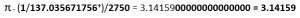In terms exclusive to Pi (π):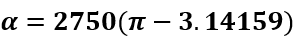Then when we divide both Pi (π) and Phi (φ) by 27.5 we get 0.114276096 and 0.0588376 respectively and adding their inverses the result is (1/0.173113696) = 5.77655, or 5777.

Moreover, Pi (π) times the Fine Structure Constant or π/137.03599… = .0229253107420, as in the ratio 27.5/12 or 2.29. Also found among the digits in this result is 253, the sum of the first 22 integers, the 22nd Triangular Number; and 107, as in the 107th Triangular Number, 5778; and 42, the Singularity. The number or ratio 229 is also the 50th Prime Number.

The reason 27.5 appears to interact flawlessly with the primordial constants of our existence and with the cosmic interface language, the Alef-bet, is that it is a frequency, actually the Primal Frequency of the Cosmos, the frequency of the aether.

And the reason that it interacts with 12 is that 27.5 is also the measure of the sacred and secret cubit spoken about in the Bible and necessary for the construction of the Tabernacle (Mishkan) of 42 elements, and the Holy Temples including the Future Holy Temple.  The sacred and secret cubit is 27.5 inches which is why is must interact seamlessly with the foot, inch, and mile, and it does.

We will explain about those interactions in great detail using the Tower of Truth as an example in a subsequent article and about the Future Holy Temple in yet another. Meanwhile, we need to explain about the frequency of 27.5, why it is unique, how it is at the core of the cosmic language of numbers, and how it is the answer to both Tesla’s statements. Afterall, what is the value of a measurement if it is not embedded with magic powers.

### The 10 Cubits of the YHVH (יהוה)

Before we begin with the cosmic language, we should note that the derivation of the sacred cubit (27.5”) also stems from the total value of the 4 aspects of the YHVH (יהוה).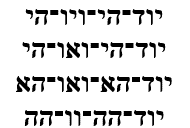The total numerical value for the 4 Aspects or 4 levels is (72 + 63 + 45 + 52) = 232. The 4 Names of the YHVH (יהוה) have 39 letters, as in the ordinal value of masveh (מסוה) to conceal—the origin of the word mask—and as in the value of “YHVH Echad (יהוה־אחד), G-d is One” from the Shema.

When we add that sum of 232 to the 39 letters in them, and to the 4 levels or 4 expanded Names we get (232 + 39 + 4) = 275. Or 10, as in the 10 sefirot (dimensions). times the 27.5” cubit and the frequency 27.5 Hz.

As we saw earlier, the 22 backs of the letters also equaled 2753. Oddly enough, like the 4 Aspects of the YHVH (יהוה), those 22 backs contain 39 letters. Moreover, the 39th Triplet of the 72 Triplets has the value of 275.

As for the 10 sefirot, the sum of the integers 1 – 10 equal 55 or (2 x 27.5), 2 cubits of 27.5” each, just like the value of the 2 letters Hei (הה) in the YHVH (יהוה) whose average is 27.5.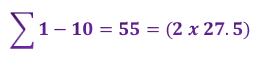# The Frequency of the Cosmos

### Let there Be Light

In the 3rd verse of the Torah, we are introduced to the concept of Light, which people take prima facia as sunlight, moonlight, starlight, but it was not until the 4th day, 13 verses later in verse 16 that the Creator made the Sun and the Moon, and it specifically says that their role shall be as a Cosmic Calendar “to serve as omens [to define] festivals, days, and years.” It says nothing about photosynthesis; the plants were functioning perfectly fine a day and paragraph earlier. It says nothing about the life-giving properties of sunlight, or that the moon is supposed to rule the tides. It is the Voice, as stated in verse 2 that moves on the water’s surface, not some magical property of the moon to attract only water and meteorites.

In the 3rd verse of the Torah, it says: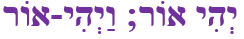The first words of G-d uttered (spoken) in the Torah are “Let there be Light (יהי־אור)” and “There was Light (יהי־אור)” located on either side of the firmament (ו) has a numerical value of 232, the same as the 4 Aspects of the YHVH (יהוה). The 13 letters in the phrase hint to One, echad, as in G-d is One. As we saw, when we add 39 for the 39 letters, as in Tal (טל), and 4 for the 4 Names within the Name’s expansion we get (232 + 39 + 4) = 275, the frequency of the Light. The complete value of the phrase is 580 with a counterspace of 420 for the 4 words, as in the 4 final letters of the phrase that also equal 420, hinting at (420 + 4) = 424, the Cosmic Consciousness as the ultimate intention of the Light/Voice at the boundary of Spherical Time.

The 4 initials of the phrase total 18, hinting at life, chai (יח), that came from His Voice, or Light, which is why the 4 letters in our DNA add up to 424.

Light or Voice has a speed, not of electromagnetic particles or waves or photons but as a perturbation in the aether. We have seen that perturbation play out as the relationship between the speed of light and the two juxtaposed components of Spherical Time, Physicality and Spirituality, also known as the metaphysical.  While the two merge together to form (3760 + 2018) = 5778, which equals precisely Phi (φ)18, the limit of the Light/Voice bubble created within the firmament, the ratio between them 3760/2018 = 186,323 mps, the Speed-of-Light in mps, off by about 42 mps from what we perceive the speed-of-light to be.

Another juxtaposition is the value (3842) of the first 42 letters of the Torah that conceal the 42-letter Name and the Cosmic Harmonic 1.273.  Through it we see that 3842/1273 equals the cube root of the 27.5, or one side of a cubic cubit (327.5), like the dimensions of the Tablets on Mt. Sinai.  The value of 327.5 is also 3.018, or One (1) plus 2.018, as in 2018 (5778 HC).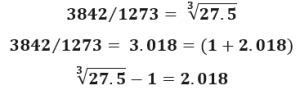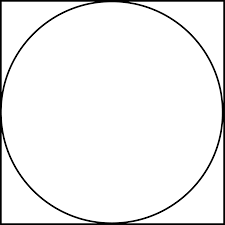Another way to view the cubic cube is as the area of a circle, 327.5.  If 327.5 is the area of a circle, then the square the inscribes that circle has an area of 1.273 or 4/π times 327.5. The resultant area of that square is 1273 327.5 = 3842, the value of the first 42 Letters of the Torah. More specifically, it is 3842.43003, adding the linked networked concepts of 424, Moshiach Ben David (Universal Consciousness), and the 3003 total of the 11 Triplets of Creation, the first 33 of the first 42 letters that total 3842.

The cosmic harmonic also is derived from the series of all odd numbers. It is a summation series were we progressively and alternately subtract and add the inverses of the odd numbers in order, they get closer and closer to π/4 whose inverse is 4/π, the cosmic harmonic (1.273…).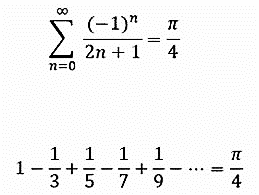Another thing about the cosmic harmonic (1.2732.…) is that when divided by 3 it equals 1.273/3 = .4244, as in Moshicah Ben David, the Cosmic Consciousness and as in the Spherical Time circumference, 36304.24470.

It is part of this cosmic alignment that 4π/3 is a key part of the equation of the volume of a sphere (4πr2/3).

### The Sphere in the Cube

We just saw the cosmic harmonic (1.273) ingrained in the 2-d relationship between a circle inscribed in a square. When we extend that to the primal 3-d relationship of a sphere inscribed in a cube, we get πs3/6 with “s” being the side of a cube.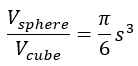Like the cosmic harmonic that is the inverse of the summation series of the set of odd numbers that equals π/4, the value π2/6 is the result of the summation series of the set of perfect squares.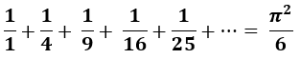The value of π/6 in the ratio between a cube and its inscribed sphere works out to .523598776…, which is off by only off by .000008022…. from .2(φ)2 or φ2/5.

Since (2.6180339887…)/5 = .523606798… Therefore: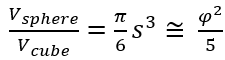This is a Primal Relationship in the expansion and contraction of the hyperspace that creates our reality as will become more apparent as we go along.

### He and His Name are One.

The 248 x 42 column/rows in the Torah less the 4 sets of 4 blank rows set between the 5 Books have a combined frequency of (248 x 4216)/27.5 = 378 like the 378-value of the Magical Essential Cube of 42, whose average value for its 9 planar tiers is 378/9 = 42 and whose average value per ordinal position is 378/27 = 14. Then when we divide the net number of rows by 27.5 squared, we get (248 x 4216)/27.52 = 378/27.5 = 13.75, as in the Phi (φ) angle (137.5o) at the center of Spherical Time. Since the resultant 13.75 = 27.5/2, the Torah Matrix or net number of rows in the Torah must equal (27.5/2 x 27.52).

Net Rows in the Torah = (248 x 4216)

(248 x 4216)/27.5 = 378

(248 x 4216)/27.52 = 378/27.5 = 13.75

Phi(φ) angle = 137.5o

Net rows in the Torah = (27.5/2 x 27.52)

Electricity, chashmol, is the same gematria as the Essential Cube of Creation, the 27th Triangular Number, 378, which is also the value of “His Name is One, Echad Ushmo Echad (אחד־ושמו־אחד).” The word Ushmo (ושמו), “His Name,” has a value of twice 173, the small gematria of the 42-Letter Name. Meanwhile, the word value 173 is found 13 times in the Torah, as in “One (Echad)” of numerical value 13. The 2 Echad (אחד) in the phrase have a collective value of 26, like the YHVH (יהוה), so altogether the phrase represents (13 + 42) + (13 + 42) = 110, as in the 110 volts in our electricity or (4 x 27.5). We will see the significance to this shortly. The only question is why Man decided to get his power from generation plants rather than the aether as Tesla had suggested.

Notably, the ordinal value of “His Name is One” echad ushmo echad (אחד־ושמו־אחד)” is 72, as in the 72 Essential Triplets, the highest Aspect of the Name YHVH (יּוד־הי־ויו־הי), and as in 72/φ2 = 27.5, while the mysterious phrase’s standard value is 378 = 27.52/2.

Meanwhile the value of the prophetic phrase is 378, which is the 27th Triangular Number, and thus the sum of the 27 integer fields, and its final letters of the 3 words spell David (דוד) of numerical value 14, which represents the 14th ordinal position at the center of the 27 positions of the Essential Cube of Creation, the Magic Cube of 42.

Setting aside that central position of 14, the complete gematria of the rest of the 26 letters is (364 + 58) = 422, the numerical value for 70, as in the 70 years of King David’s life at the center of Spherical Time. With the kolel, it is 424, Moshiach Ben David, the Cosmic Consciousness at the boundary of Spherical Time.

“His Name is One,” just like Phi (φ):### The First 42 Letters and 27.5

Within those special first 42 letters of the Torah the ordinal positions (27th and 28th) are the final two letters (רץ) of the Torah’s first verse. The other 14 letters begin the 2nd verse.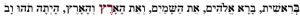These are also the middle two letters (רצ) of the 42-Letter Name Matrix. They add up to (27 + 28) = 55, an odd Pronic Number that equals 2 cubits (2 x 27.5). Out of the 210 word values in the Torah, the word value 27 is found 83 times and the word value 28 is found 82 times giving us the simple equation (27 + 83) = (28 + 82) = 110 = 4 x 27.5.

The Pronic set of (27 and 28) has a product of 756 and a sum of 55, making its P/S cipher equal to 756/55 = 13.75, the Phi (φ) angle (137.5o) and half of 27.5, which is the average of the two.

Their recurrence Pronic counterparts (82 and 83) sum to 165 or (6 x 27.5) and their P/S is 41.248… alluding to 248, the numerical value of the Name Abraham, and 41 the ordinal value of Abraham. Moreover, while 412 is the value of the Name of the Torah’s first letter (בית), it is also (3 x 137.5o) and thus 3.000… times that other P/S ratio 756/55.

And while (27 + 28) + (82 + 83) must equal (8 x 27.5), the sum of their products (27 x 28) + (82 x 83) = 7562 or 275 x 27.5. = (10 x 27.52).

The 27th and 28th letters (רץ) of the Torah’s first verse convert into the letters (בט) within their corresponding 27th and 28th positions in the 42-Letter Name Matrix. These 2 letters (בט) have the numerical value 11 or (27 + 28)/5. As we are about to see this is not coincidental.

### The Network of 210 Word Values

When ranked in order of abundance, the 210 word values in the Torah that form a 322 Matrix that folds up into a hypercube, reveal interesting patterns. This 322 Matrix overlaps with the hyper network of the exact 81,000 words and word values in the Torah, a (90 x 900) matrix or (90 x 90 x 10) array whose 6th root is 6.5778026, as in the Event Horizon of the YHVH (יהוה) in the 6th millennium, just like the log of the 600,000 Israelite Men, 5.778

The top word value in the Torah in terms of recurrences is 401 that is found 2669 times for a total value of 1070269, as in the 107th Triangular Number, 5778 and the 26 of the YHVH (יהוה) again. It represents 3.3373% of the words in the Torah, like the ratio of the Sun to the Earth, etc., while the sum of its value, recurrences, and total value is 1073339, as in 57782 or (5778 x .5778) that equals 3338.5 or 3339, as in the year the First Holy Temple was destroyed.

The top 8 values in terms of their Torah recurrences stretch from the value 401 to 407, which are the values of the Torah’s 4th and 6th words respectively, (את) and (ואת), whose joint value 808, is that of Abraham, and whose joint complete value is (424 + 436) = 860 which equals 10 times the 86-value of Elohim (אלהים), the Torah’s 3rd word.

The total gematria value of the entire Torah is 21009826 and when divided by the YHVH (יהוה) or alternatively the Great Precession we get 21009826/26000 = 808.0702, giving us that same 808, along with 702, the value for Shabbat.

The total gematria value of the entire Torah, 21009826, begins with 21, as in the Name Ehyeh (אהיה), and ends with 26, as in the YHVH (יהוה) with 98 attached to the 26, as in the 98-value of the 5 prefixes of the YHVH (יהוה). The total value of the 294 prefixes used in conjunction with the YHVH (יהוה) is (294 + 98 + 7966) = 8358, as in the 8000 or 203 cu3 Foundation Block of the 72 Triplets (Names) and the Holy of Holies in the Holy Temple, coupled with 358, Moshiach. It is also (200 x 4242). It is also the first 203 cu3 tier of the 210-cubit tall Tower of Truth.

Those top 8 values sum to 13,911 occurrences, as in the Path of One from 11391… and as in the 911 paragraphs, chapters, and portions in the Torah. These top 8 recurrence figures represent 17.3…% of the words in the Torah, as in the 173 small gematria value of the 42-Letter Name, which the ancient Zohar refers to as the 173 Keys to Heaven given to Moses.

The 20th word value in terms of recurrences is 37 found 555 times in the Torah. The top 20-word values, representing about 2.0% of the total 210 word values, and as in the 20 stages of the Tower of Truth, etc. sum to 22,006 occurrences, which is 27.5% of all the words in the Torah, as in the Primal Frequency (27.5 Hz) and the cubit (27.5”) used to construct the 210 cubit high Tower. Those 22,006 occurrences divided by 27.5 is 800.22.

## The Cosmic Frequency

### The Magic Squares and CubesThe second line/level of the 42-Letter Name Matrix (קרעשטן) has a value of 729 or 9 x 9 x 9 or 272 as in the 9-planar structure of the 27 positions of the Essential Cube of Creation that equals 42 and as in the (9 + 9 + 9) exponential tiered structure of the 27 letters of the Alef-bet.  This is in line with the 72 verses in the first 9 paragraphs in the Torah and the 9 rows in the 72 Triplet Matrix from the Torah.

While those 6 letters (קרעשטן) using small gematria form the numeric string 127395, as in the cosmic harmonic 1.273, they also form a numeric anagram of 913275, that translates to “In the Beginning, the sacred frequency (27.5).”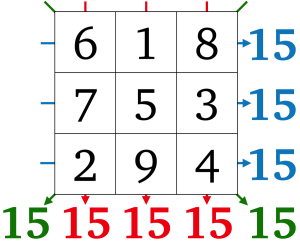The Magic square of the integers 19 sums to 15 in each direction including its diagonals, as in the value of the Name Yah (יה).  This is a “normal” magic square because its positive integers are in sequential order starting with 1.

While this 32 normal magic square yields the number 15; the 42 one yields 34; the 52 one yields 65, as in Adonai (אדני); and the 62 one yields 111, as in Alef (אלף); and together these first 4 with their (9 + 16 + 25 + 36) = 86 total component squares, as in Elohim (אלהים), yield a total of (15 + 34 + 65 + 111) = 225 or 152.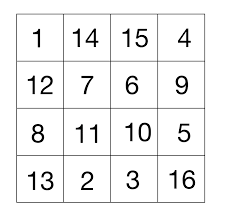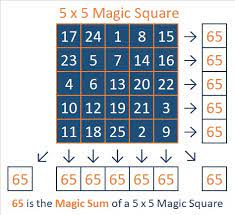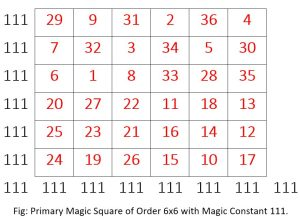## The Unfolding of Creation

### The Verses, Frequency, and Pattern of Creation

That the normal 42 magic square that yields the constant 34 connects with the 34 verses in Creation, which in turn connects with the Fibonacci Numbers (0, 1, 1, 2, 3, 5, 8, 13, 21, 34) that are based on Phi (φ).

The 1st Day of Creation ends on verse #5; the 2nd Day on verse #8; the 3rd Day on verse 13; and the 4th, 5th, 6th and 7th Days contain 21 verses and end on the 34th verse in the Torah. Of those the 4th and 5th Days end on verses 19 and 23 or together (19 + 23) = 42, while the 6th Day ends on 31, making (42 + 31) = 73, as in the Triangular value of the Torah’s first verse (2701) that is 73% of the value of the 42-Letter Name (3701), and as in the Fine Structure Constant (1/137 or .0073). Each subsequent number in the Fibonacci Sequence is a multiple of Phi (1.618033..) more than the next. Also, those first 3 days that end on verses, 5, 8 and 13 add up to 26.

The simplest pattern of Creation is the 1st Day is 5 verses; the 2nd and 3rd Days are 8 verses, summing to 13 so far; and the 4th through 7th Day is 21 verses, or 34 in total 58132134 verses. The 1st Day consists of the 2 first singular verses that are integrally connected, followed by 3 verses about the Light, therefore the full pattern is 0, 1, 1, 2, 3, 5, 8, 13, 21, 34.

The 1st plus 2nd plus 3rd plus 4th plus 5th verses of the 1st Day of Creation add up to (1 + 2 + 3 + 4 + 5) = 15, as in constant yielded by the 1st normal magic square. The reason those yielded constants from the normal magic squares are significant is that they are the emanation values from which the magic squares were projected, in the same way that the Essential Cube of Creation was emanated from the Source or Singularity of 42.

Normally emanations are radial and even, such as 3 radiating to 32 or 9, or to 33 or 27 equal. Their spacing and positioning is even, but with the magic squares and the magic cube they are projected and filtered according to a very specific order. And through analyzing that order we can learn more about the Source emanation.

The 2nd Day is comprised of the 3 verses (6th + 7th + 8th) that equals 21. While 15 was naturally the 5th Triangular Number corresponding to its 5 verses, as in the 5th Fibonacci Number (5), the 2nd Day’s (68) verses equal the 6th Triangular Number and the 8th Fibonacci Number (21).

The 3rd Day is comprised of the 5 verses (9th + 10th + 11th + 12th + 13th) that equals 55, the 10th Fibonacci Number and (2 x 27.5).

The 4th Day is comprised of the 6 verses (14th + 15th + 16th + 17th + 18th + 19th) that equals 99, as in (3 x 33), whose average is 16.5 or (.6 x 27.5).

The 5th Day on the other hand is comprised of 4 verses (20th + 21st + 22nd + 23rd) that equal 86, as in the value of the Name Elohim (אלהים). Earlier we saw that the 4th and 5th Days together equaled 42, and now we see that their (99 + 86) verse totals equal 185, which the Arizal explained is the value of the Name El (אל) spelled-out (אלף־למד). It is also cosmically designed as the first two letters in Elohim (אלהים), whose last 3 letters (הים) equal 55.

They are also the first two letters of the Name of 42, Eloah (אלוה). My Rav always said “what is less is more” when it comes to Torah study, and the Name of 42, Eloah (אלוה) is found only once in the Torah, the only singular Name found only once.

The 6th Day is comprised of the 8 verses (23rd + 24th + 25th + 26th + 27th + 28th + 29th + 30th + 31st) that equals 220, as in (8 x 27.5) or an average of 27.5 per verse. Thus the 3rd and 6th Days = (55 + 220) = 275.

The 7th Day is comprised of the 3 verses (32nd + 33rd + 34th) ending on the 9th Fibonacci Number (34) and equaling 69, which we will soon see is connected to the powerful 6 x 6 normal magic square. While the verse total sum for the first 6 Days is (15 + 21 + 55 + 99 + 86 + 220) = 496, the 31st Triangular Number, it is also the 3rd Perfect Number, in the same way that 6 is the 1st Perfect Number. Meanwhile, the sum of the square roots of the 7 Days verse totals is 30.5777.

The verse total sum of the 7 Days (15 + 21 + 55 + 99 + 86 + 220 + 69) = 565, and the sum of the ending verses for the 7 Days is (5 + 8 + 13 + 19 + 23 + 31 + 34) = 133, leaving them a difference of (565133) = 432, as in the 432 HZ frequency of Binah and the Sun’s radius (432,000 mi).

There is no 2 x 2 normal magic square and we do not count 11; nevertheless (11 + 22 + 32 + 42 + 52 + 62) = 91, as in the 13th Triangular Number in the Path of One (113914551820). As Moshe showed me, this is also like the 26 different verses comprised of 42 or 16 letters and the 65 different verses comprised of 52 or 25 letters, which together equal (26 + 65) verses, exactly like the values of the Names YHVH (יהוה) and Adonai (אדני). Moreover, there are 86 verses comprised of 62 or 36 letters, as in the Name Elohim (אלהים), whose ordinal value is 41, as in the (42 + 52) letters. Together the (42 + 52 + 62) letters equal 77, as in the sum of the Torah’s first 8 words or 33 letters, 3003, that is the 77th Triangular Number, and that form the 11 Triplets of Creation. The odds against this happening coincidentally by random chance are astronomical, so the question is why are the normal magic squares aligned with the (183 + 13) verses in the Torah?

And why these have to align with the sum of the integers from 26 to 65 that are equal to 1820, as in the 1820 YHVH (יהוה) in the Torah in the Path of One?

As for the rest of Bereshit, Moshe also helped me see that after the initial 34 verses in the Creation story, there are 112 in the subsequent 22 chapters of the Torah’s first portion, Bereshit, as in the 112 Triplets concealed in the Torah.  After the 34 verses in first 7 Paragraphs of the Torah, the 112 verses are broken down to the 86 verses in the 8th16th Paragraphs and the 26 in the 7 final Paragraphs of the Torah’s first portion, mimicking and aligning with the unification of Elohim (אלהים) and the YHVH (יהוה) or (86 + 26 = 112), and the 2 axes of the 112 Triplets (14 + 72) and (11 + 15). Those final 26 verses are further broken down to 13 and 13 for the 17th – 20th Paragraphs and the 21st – 23rd ones. The 86 verses are likewise split evenly in half according to their first 3 and later 6 Paragraphs. We see a similar pattern in the 86 verses that stretch from the 26th Paragraph to the end or parsha Noach.

Meanwhile, like the verses in the 7 Days of Creation, the verses in parsha Noach also follow the Fibonacci Number pattern with the verses split into 55, 89, and 144 verse Phi (φ) proportions. The 24 – 39 Paragraphs equal 144 verses, while the 24th – 28th Paragraphs equal 89 verses and the 29th – 39th Paragraphs equal 55 verses. Those 55 verses are further split into 21 and 34 verses in terms of their Paragraphs.

Over the 299 verses through the first two portions and the 41st Paragraph, they are divided into the Phi (φ) proportion at the 15th Paragraph as in (15 + 26) = 41, the ordinal value of Elohim (אלהים), and as in YH (יה) and YHVH (יהוה). The sum of the integers of 15 through 41 is 756 or 27.52 and the numerical value of “sefirot (ספירות)meaning dimensions or spheres, referring to the Tree-of-life.

The 3rd portion Lecha Lecha stretches from the 42nd to 48th Paragraphs and contains 126 verses or (3 x 42), like the 126 verses through the first 18 Paragraphs of the Torah, and like the 126-value of the faces and planes of the Essential Magic Cube of Creation. This is 300 verses before the end of Lecha Lecha and the start of the 42nd Paragraph. Since 300 is the 24th Triangular Number, when we add it to 18, we get 42.

It goes on and on, including the 275 verses from the start of the Flood reset in paragraph 25 to the end of parsha Lech Lecha when Abraham, Isaac, and every man in their household was circumcised. Then G-d appeared to Abraham to begin Chapter 18.

Over and over again, we see the importance of the deliberate parsing and design of the Torah in terms of its 670 Paragraphs and let us not forget that these multi-level alignments and networked connections are all arranged within a 183 cubic array.

### The Mystical 6 x 6 Magic Square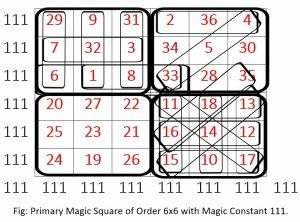Meanwhile, there are some very special properties about this 6 x 6 normal magic square of Alef (אלף), 111, or “One” that aligns with the first 111 verses in the Torah through the first 13 Chapters, as in the value 13, echad, also “One.”

While every vertical, horizontal and diagonal line equals 111, in each of them there is a sequential 3 number sequence at the beginning or end—with one exception in the middle—that adds up to 42. This split of 111 into 42 and 69 splits it into the 42/111 = .378378 proportion and the difference between them is (69 42) = 27, as in the 27 positions of the Essential Cube of Creation that collectively add up to 378 and whose verticals, horizontals, and diagonals equal 42.

This also connects with the 69-value of the 3 verses of the 7th Day of Creation.

Moreover, within the extraordinary 6 x 6 normal magic square we see find 1/Phi (φ) or .618033 represented as 61833 in the 3rd row, and Phi (φ) or 1.61803 as 16183 in one of the highlighted diagonals.

Furthermore, the bottom right quadrant forms a perfect 3 x 3 magic square of 42, while the upper left quadrant forms a square of (3 x 42) or 126, as in every face and plane of the Essential Cube of Creation.  Their complementary quadrants likewise form a perfect 3 x 3 magic square of 69 (bottom left) and a square (upper right) of 3 x 69 or 207, as in the value of Light, Ohr (אור). Together each complementary pair of quadrants equals (126 + 207) = 333, while the entire matrix equals 666.

### Portions and Paragraphs

That makes sense since the sum of the integers from 136 is 666, and yet what is intriguing is that this equals the sum of the starting Paragraphs for the 12 Torah portions in Genesis (Bereshit): 1st + 24th + 42nd + 49th + 55th + 59th + 63rd + 64th + 73rd + 77th +78th + 81st = 666, the 36th Triangular Number.

The 10th portion contains only the 77th Paragraph, as in the 77th Triangular Number 3003 that is the value of the Torah’s first 8 words and/or 33 letters that make us the 11 Triplets of Bereshit. Of course, the previous or 9th portion begins with the 73rd Paragraph, as in the 73rd Triangular Number, which is the value of the first 7 words or first verse of the Torah.

There are 146 verses in that first portion of the Torah and 146 is twice 73, and within this set of 12 portions the 10th portion (Miketz) also has 146 or (2 x 73) verses. Like the 2 sets of 2 dreams and 2 years in Miketz and 2 juxtaposed paradigms in Bereshit, perhaps the Creator was hinting at the 2 worlds or 2 universes, seeing as 146 is the numerical value of Olam, (עולם), meaning the world or universe.  And another clue can be found when He made the ordinal value of all the initials in all 12 portions equal to 146, and their complete value, 1042.

He also designed the Torah so that there would be 54 portions in all 5 Books, and 12 in the first Book, leaving 42 in the rest, similar to the equation we saw earlier, √42/12 = 54.

The last 9 initials of the 12 Portions that equal 784, or 282 after the first 3 (בנלל) that equal 112, as in the 112 Essential Triplets of the Torah, like the 112 verses in the 9th Portion.

As for the other portions in this first set or network of 12, the 5th one, Chayei Sarah begins with the 55th Paragraph as in the 10th Triangular Number and 10th Fibonacci Number; the 4th begins with the 49th or 72 Paragraph; the 8th portion that begins with Paragraph 64 and ends with Paragraph 72 for a total of 9 Paragraphs reflects the 8 x 9 matrix and 64-square grid within the layout of the 72 Triplets; the 11th Portion begins with the 78th Paragraph, as in the 12th Triangular Number; while the 12th Portion begins with the 81st Paragraph, as in 92.

Moreover, the middle 6 Portions (49 + 55 + 59 + 63 + 64 + 73) = 363, as in H’Moshiach, the Cosmic Consciousness, and the Spherical Time circumference (36304.24470). The beginning 3 portions and the final 3 Portions of this set of 12 must then add up to 303 for their starting paragraphs. And their corresponding ending paragraphs (23 + 41 + 48) = 112 and (77 + 80 + 91) = 248, as in the 112 Triplets concealed in the Torah and 248 dimensions, etc., which together equal (112 + 248) = 360, while the middle 6 equal 385, as in “the Divine Presence, the Shechinah (שכינה),” and the difference between those 6 start and ending verses is (385363) = 22, as in the 22 Letters and sum of the 22 integers between 728 that sum to 385. Time to circle back to the 6 x 6 magic square.

### Back to the Other MagicI am sure there is lots more to be found in this 6 x 6 normal magic square, like the crisscrossing diagonal digits in the upper right quadrant forming 253 and 354 as in the sum of the 22 integers (253) and the number of days in the lunar calendar (354). Meanwhile the value of each of the diagonals in that quadrant is 42.

There is also the bottom right perfect 3 x 3 magic square of 42, whose first digits in each box (position) form 111, 111, 111 and whose second digits form 0, 1, 2, 3, 4, 5, 6, 7, 8, while the top left boxes form 1118, the value of the first verse of the Shema and the concealed 15 Triplets within it.

And like the normal Magical Essential Cube of Creation whose central position is 14, the 4 central digits in this 6 x 6 normal magic square equal (8 + 2 + 3 + 1) = 14.

If we examine the various corners and middle positions throughout this 6 x 6 or 36 position normal magic square, we will find they all total 74 and since (111 74) = 37 and 111 = (3 x 37) we see them once again partitioning the square into the .333 and the .666 proportion. We are reminded of the Torah’s first verse whose value is (37 x 73), the 73rd Triangular Number, and whose value of its last two words is 703, the 37th Triangular number, which means that its first five words have the value 1998 or (18 x 111), the 36th Triangular Number times 3, as in (36 + 37) = 73. Moreover, the 1998-portion is 74% of 2701, and the 703-portion is thus 26.0% as in the YHVH (יהוה) and the 260-constant yielded by the 8 x 8 normal magic cube.

While the value of the Torah’s first verse 2701 = (37 x 73), and the numbers 37 and 73 together equal (4 x 27.5), they also represent the 12th and 21st Prime Numbers respectively, and while (21 + 21) = 33, the 33rd Prime Number is 137, as in the Fine Structure Constant. Meanwhile, (12 x 21) could represent 12 Ehyeh (אהיה) which also equals (6 x 42).

We must keep in mind that man did not create this matrix. Man just finds or stumbles upon them. Man does not create numbers or the patterns, sequences, or constants they make. Neither could aliens, subterranean terrestrial bound ones, or even fictitious out-of-space ones. Only the Cosmos can do that and the One who created the Cosmos, whether we want to call Him by the Name Nature, Elohim (אלהים), or by the Name YHVH (יהוה).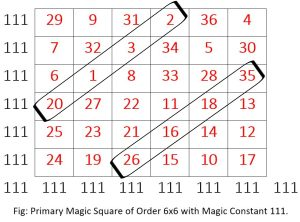Another pattern discernible in the 6 x 6 normal magic cube are the parallel 4-number diagonal sequences that add up to 26 and 95 respectively, as in H’Melech YHVH (המלך־יהוה) or God, the King, with the second sequence beginning with 26 and including Phi (1618). Together, their value is 112.

### The Magical 8 x 8 Square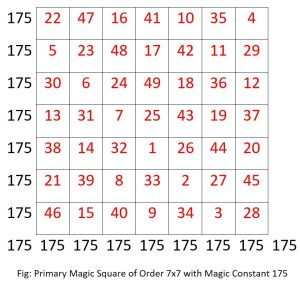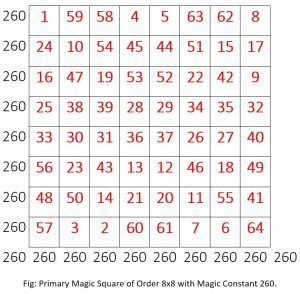Next, we have the 7 x 7 normal magic square that yields 175, as in the 175-year life of Abraham, while the 82 one yields 260, making the 32 and the 82 ones together total (15 + 260) = 275, the sacred cubit and frequency, while (3 + 8) = 11, which is integrally related to the Primal Frequency 27.5 as we shall soon see.

The 82 magic square matches the 82 Triplets in the Foundation Block of the 72 Triplets that together have a value of 8000 or 203. But the similarity is far deeper than that because the 4 numbers at the center of the 82 magic square (28, 29, 36, 37) match exactly in the exact same order as the ordinal positions of the 4 Triplets at the center of the 72 Triplets (28th, 29th, 36th and 37th).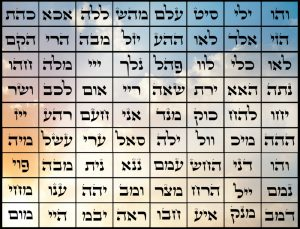The initials (שרמא) of those 4 central Triplets equal 541, the value of Israel, and their ordinal value is 55 or (2 x 27.5). The second letter in each of those 4 Triplets that are also known as the Alchemist Stone sum to 111, as in the constant yielded by the 6 x 6 Magic Cube. This only happens if they were designed that way. The question again is why?

Those same 4 central numbers add up to 130, as in Sinai and half of the 260 line total, while the 4 corners (1, 57, 8, 64) also add up to 130, as do the opposite bookends of the central 4: (25 + 33 + 32 + 40) and (4 + 5 + 60 + 61). There are also numerous quadrants that add up to 130 and pairs of quadrants that add up to 260, giving them an average of 130. It was also in the 130th verse of the Torah when Enoch walked with G-d, a hint to when Moses would walk with G-d on Mt Sinai.

### Magic Cubes in the Order of 8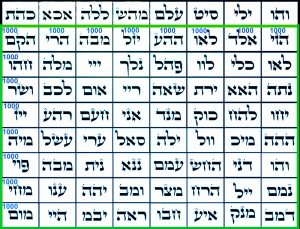That Foundation Block in the 72 Triplets divides the 8 Triplets in the upper row from the 64 below. The value of those 64 or 82 is exactly 8000 or an average of 1000 per row or column. Since the top row of 8 equals 1143, the total value of the 72 Triplets is 9143, and while the average of that top row is 1143/8 = 143, the value 1143 is exactly 1/8th of 9143, and the exactly 1/7th of 8000. This is the real Magic Square, or even cube of 63 letters.

As it turns out all Special Magic Cubes are in the order of 8.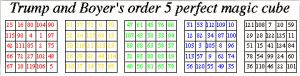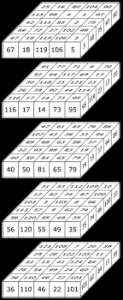A Magic Cube is Perfect if besides all axial equal sums the diagonals, both facial and internal, are equal as well to the Magic Constant n(n3 + 1)/2. There are not many of them. Trump and Boyer found the 5th order (53) and the 6th order (63) ones. There are none less. Interestingly from a Cosmic perspective the 5th order one has a central number of 63 and magic constant of 315, which together equal 378, just like the total of the Magic Cube of 42. This is also like the 378 verses in the 3 consecutive portions of the Torah (Lech Lecha, Vayeira, and Chayei Sarah). The 6th order magic cube has no central value—no even number one can—and its magic constant is 651. Together (42 + 315 + 651) = 1008.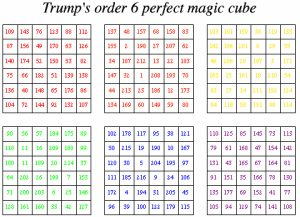This may or may not be related to, but certainly is aligned with 10080 miles of the Earth and Moon diameters, and the 10080 minutes = (7 days x 24 hours x 60 minutes) in 7 days, which in turn is aligned with the Earth and Moon radii, 5040 miles = 7! or (7 x 6 x 5 x 4 x 3 x 2 x 1). A further alignment is also that 1008 is also the 7th Triangular Number times the 8th Triangular Number or (28 x 36), and the sum of the middle pair, Darosh Darash (דרש־דרש), of the 90 doubled words in the Torah. While the sums of the diameters of the Earth and Moon together, 10080 miles = 240 x 42, and they are 240,000 miles apart or 112 Lunar diameters from their centers, the ratio of 10080/112 = 90, as in the 90 doubled words in the Torah, and the 112 Triplets concealed in the Torah.

As for the distance between the Earth and the Moon of 240,000 miles, and the Earth and Moon diameters of 10,080 miles, as in the atomic weight (1.0080) of Hydrogen, which makes up almost 75% of the mass of the universe, the ratio between their size and their distance apart is thus 10,080/240,000 = .042.

Beyond the perfect magic cubes there are also bimagic and trimagic cubes whereby their principles of a magic cube must hold for their values squared and cubed respectively. The smallest known bimagic cube is of order 16 or (2 x 8); the smallest known perfect bimagic cube is of order 32 or (4 x 8); the smallest known trimagic cube is of order 64 or (8 x 8), and the smallest known perfect trimagic cube is of order 256 or (32 x 8). All the special magic cubes are in the order of 8.

### The Frequency in the 3 x 3 Magic Square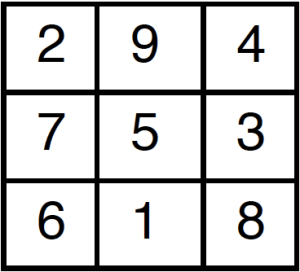Nonetheless, that first 3 x 3 or n = 9 normal magic square yields 15 and thus aligns with the equation 171.313 / 27.5 = 1.500, YH (יה), which is also the highest word value in the Torah (1500). And while it is also the value of the product (1500) of the letters in the YHVH (יהוה), we find it in all the components of the story of the Flood (15 cubits of water, 150 days, 150 days again, and the product of the dimensions of the ark, (1500).

The first paragraph of parsha Noach is paragraph 24 and while 300 is the 24th Triangular Number, this paragraph ends on verse 150 with the beginning of the Great Flood and there are 150 verses more until the end of the portion and the 42nd Paragraph.

This first magic square is special in yet another way. While one of its rows forms the numeric string 618 as in 1/Phi (φ), the sum of its 3 rows (294 + 753 + 618) equals the sum of its 3 columns (438, 951, and 276) and they each equal 1665 or (15 x 111), which is 1/3rd the 4995 values of the 27 or 33 letters of the Alef-bet.

Of course, the sum of both of those rows and columns together equal 3330, as in all those Cosmic ratios within our Solar System, and as in the 3330 years from the reception of the Torah at Mt Sinai and the Event Horizon in 5778 HC.

This is also like the first 15 letters of the Torah whose value equals exactly 1500 and is aligned with the 1500 verses from the End of the 7th Day of Creation to the end of the Book of Genesis (Bereshit). Of those 15 letters, the first 9 have a collective ordinal value of 99 and the final 6 have a collective ordinal value of 42, which is like the 3 tiers of 9 letters that equal 42 in all direction and whose total is 378/9 = 42.

As we have previously explained, the numerical values of the 27 letters of the Alef-bet are based on a simple exponential equation and a sine wave that rides astride it, yet they are also 3 parallel groups of 9: 1 – 9, 10 – 90, and 100 – 900. It is because of that structure that the 27 letters sum to 4995. There is another way to get to 4995 though, and it involves the uniqueness of the sacred cubit (27.5”) and its associated frequency (27.5 Hz).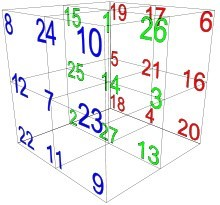What is astonishing though is that while the 32 normal magic square surreptitiously gives us this value of the 1665 or (15 x 111) that aligns with YH (יה) x Alef (אלף), representing the yield values of the 1st and 4th such magic squares, the 33 normal magic cube is divided into 3 horizontal planes that each total 1665 when the 33 values of the Alef-bet are applied to them.  Moreover, the 3 rows (618, 753, and 294) of the square are each found on 3 different tiers or vertical planes of the cube.

The first normal magic square of 3 x 3 has one of its rows forming the numeric string 618 as in 1/Phi (φ), matching the diagonal (618)of the top 3 x 3 face/tier of the normal Magical Essential Cube of Creation. When the Hebrew letter interface is applied to the 9 squares, the 3 rows (816, 681, and 168) form a new semi-magic 3 x 3 square which like the normal 3 x 3 magic square somehow has a constant of 15, and it also has a column of (618) or 1/Phi (φ).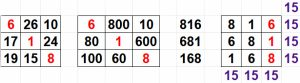Likewise, the middle tier of the normal Magical Essential Cube of Creation forms 3 rows (573, 357, and 735) form a new magic 3 x 3 square this time, which like the normal 3 x 3 magic square somehow has a constant of 15, and it also has a middle column of (753).

Likewise, the bottom tier of the normal Magical Essential Cube of Creation forms 3 rows (249, 924, and 492) form a new semi-magic 3 x 3 square, which like the normal 3 x 3 magic square somehow has a constant of 15, and it also has a first column of (294).

We can see the magical dynamic at play, whereby the normal magic 32 square of constant 15 unfolded into the 33 normal magic cube of 42, the Essential Cube of Creation.  This is like the initials of 42, MB (מב) that have the standard value 42 and the ordinal value 15.

While we covered 618 from the 3 rows (618, 753, and 294) of the magic square, the value 294 may be significance too because of the 1820 YHVH (יהוה) in the Torah, there are 294 with prefixes, and that is .1618 or Phi (φ) percent of 1820.

The value 294 is also (7 x 42), while 1820/7 = 260, as in the constant yielded by the 82 normal magic square. While 7 may refer to the 7 higher Names Ehyeh (אהיה) in the Torah and the level of Binah, the Name Ehyeh (אהיה) has a value of 21 so conversely 294/21 = 14, as in the central position in the 33 normal Magical Cube of Creation (42).

Kabbalists, alchemists, magicians, mathematicians, and philosophers from time immemorial have studied the intrigues of magic squares, searching for insights into the mystical and metaphysical boundaries of the universe. By pairing the 32 normal magic square of 15 with 33 normal magic cube of 42, the Creator has proven the existence of the Essential Cube of Creation with its cosmic interface between the 27 letters and the 27 integers or ordinal values.  And we also see that (15 + 27) = 42.

Besides the Essential Cube of Creation, there are 3 other normal magic cubes of the order 33 and all add up to 42 as their constant with position 14 in their center, and as such they all have the same core dynamics and all have the same 26 vectors with at least 2 axis vectors in common, though their arrangement is somewhat varied.

What else is interesting and only makes sense with an understanding of hyperdimensional mathematics and the Cosmic Wheel is that the diagonal of the 32 normal magic square is 258, as in the (42 + 216) days until the Day of Creation, and that the cumulative difference in the yield (i.e. 3415) values for the first 6 normal magic squares is (19 + 31 + 46 + 64 + 85 + 109) = 354, the lunar year again.  Moreover, those 9 positions that equal 1665 in both the 32 normal magic square of 15 and the 33 normal magic cube of 42 when subtracted from 1665 equal (16659) = 1656, the year of the Flood, 1656 HC, exactly 4125 years ago, or 150 x 27.5 years, 3 times the Phi (φ) angle (137.5o).

Nikola Tesla was obsessed with the number 3 and subsequently with 9 and 18.  He was also obsessed with frequencies. So were the ancient kabbalists.  The number 27.5 is a very special number, unique in how it interacts with the number 9. At its core though it is a frequency that ties together all the other numbers.

“…if you knew the magnificence of 3, 6 and 9, you would have a key to the universe.”The 42-Letter Name begins just like the Alef-bet or Cosmic Interface with the 3 letters Alef (א), Bet (ב), and Gimmel (ג) of numerical values 1, 2, and 3. That Triplet (אבג) is found only 3 times in the Torah, all in portion Mishpatim. Tesla knew that 3, 6, and 9 related to frequency and vibrations.  We know that the Singularity of the Cosmos is 42 and anyone who understood the secrets of the universe would have as well. What Telsa knew was that: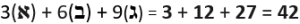This is just the beginning.  Part 2 follows shortly….